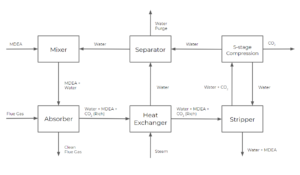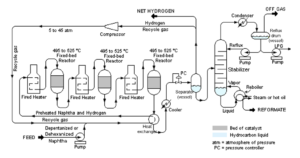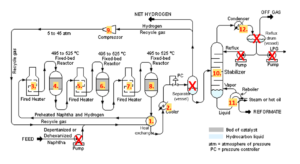# 51 Practice Exercises

## Multiple Choice Questions

Exercise: Equipment Prices with the Effect of Capacity and Time

Equipment Type Range of Correlation Capacity Units Cost Exponent (n)
Air compressor, multiple stages 1 -1500 $kW$ 0.85
Shell and tube heat exchanger stainless steel 1.9 – 1860 $m^2$ 0.60
Horizontal tank carbon steel 0.5-74 $m^3$ 0.30
centrifugal pump stainless steel 1-70 $hp$ 0.67
Crystalizer 0.2-3.8 $m^3$ 0.47
Year Marshall and Swift Equipment cost index Chemical Engineering Plant Cost Index
1996 211.7 154.7
2000 264.8 169.3
2004 301.7 186.3
2008 339.2 212.8
2012 391.5 227.8
2016 418.9 237.8
2020 458.3 258.8

In 2008, a carbon steel horizontal tank with a capacity of 26.5$m^3$ gallons costs $6700, then which of the following is the closest estimation to the cost of the same type of tank with a capacity of 50$m^3$ cost in 2020? a)$9800

b)$10,100 c)$7,500

d)$8,000 ### Solution b)$10,100

We combine the effect of capacity and time by multiplying the two factors. For time, we use the Marshall and Swift Equipment cost index because we are interested in the cost of a piece of equipment.

\begin{align*}
C_{2} &=C_{1}×\Big(\frac{A_{a}}{A_{b}}\Big)^n×\Big(\frac{I_{2}}{I_{1}}\Big)\\
C_{2} & = $6700×\Big(\frac{50}{26.5}\Big)^{0.30}×\Big(\frac{458.3}{339.2}\Big)\\ C_{2} & ≈$10,100
\end{align*}

Exercise: Preliminary Estimation of Purchased Equipment Costs

Using the preliminary estimation of purchased equipment costs, estimate the price of a centrifugal compressor with the power of 400kW.

The value of a, b and n are shown as follows:

 Equipment Units for Size, S S lower S upper a b n centrifugal compressors driver power, kW 75 30,000 490,000 16,800 0.6

a) $1,100,000 b)$7,210,000

c) $490,000 d) the size of the equipment is out of the range for effective estimation ### Solution a)$1,100,000

The size of the compressor is 400kW, which is within the range of S lower and S upper, so we can eliminate choice d).
Then we can use the formula:

\begin{align*}
C_e&=a+bS^n\\
&=490,000+16,800×(400)^{0.6}\\
&≈ 1,100,000 \end{align*} Exercise: Calculating Depreciation  Equipment A Equipment B Purchased Value80,000 $100,000 Salvage Value$30,000 40,000 Life of Equipment 5 years 10 years Suppose we have 2 choices for a piece of equipment that serves the same purpose in our process, and we want to choose the equipment with the smallest depreciation, which one should we choose? a) equipment A b) equipment B c) they have the same depreciation value ### Solution b) equipment B \begin{align*} &\text{Depreciation of equipment A}\\ &=\frac{\text{purchased value}-\text{salvage value}}{\text{life of equipment }(n)}\\ &=\frac{80,000-$30,000}{5years}\\ &=$10000/year
\end{align*}

\begin{align*}
&\text{Depreciation of equipment B}\\
&=\frac{\text{purchased value}-\text{salvage value}}{\text{life of equipment }(n)}\\
&=\frac{$100,000-$40,000}{10years}\\
&=6000/year \end{align*} ## Long Answer Questions Exercise: Estimating Project Cost Using the Lang FactorShown above is the block flow diagram of a carbon-capture process. The cost of equipment for each step is listed as follows:  Equipment Price Absorber80,000 Heat Exchanger $65,000 Stripper$50,000 5-stage Compression $120,000 Separator$30,000 Mixer 30,000  Type of Plant FCI TCI solid processing plant 3.9 4.6 solid-fluid processing plant 4.1 4.9 fluid processing plant 4.8 5.7 All of the compounds are in liquid or vapour states. Estimate the TCI of the project. ### Solution Since all of the compounds are in liquid or vapour states, we take $F_{lang}=5.7$ \begin{align*} C_{TM}&=F_{lang} \sum_{i=1}^{n}C_{p,i}\\ &=5.7×(80,000+$65,000+$50,000+$120,000\\ &\;\;\;\;\;\;+$30,000+$30,000)\\ &=$2,137,500
\end{align*}

Exercise: Calculating the number of Operating Labour

Shown below is a PFD of a catalytic reforming plant used in oil refineries to produce a high-octane gasoline component. Calculate the number of operators needed per shift for the process.Image obtained from User: mbeychok via Wikimedia Commons / CC BY-SA 3.0

Note: Naphtha is a flammable liquid hydrocarbon mixture

### Solution

From the labels, the process does not involve handling solids($P=0$). And we can count that we have a total of 12 process steps that handle fluids without accounting for the number of pumps and vessels, shown in the diagram below:So $P=0\;;N_{np}=12$. Substitute this into the formula:

\begin{align*}
N_{OL}&=(6.29+31.7×(0)^2+0.23×12)^{0.5}\\
& = 3.008\\
& ≈ 4
\end{align*}

The number of operating labour is rounded up to the nearest integer.

Exercise: Return on Investment

Suppose the estimation of the key manufacturing costs for our process are listed below:

 $FCI$ $1,650,000 $TCI$$2,000,000 $C_{RM}$ $600,000 $C_{UT}$$280,000 $C_{OL}$ $320,000 $C_{WT}$$500,000

a) Calculate the cost of manufacturing (do not include depreciation)

b) If the market price of the product is 500/tonne, how many tonnes of the product do we need to sell per year to get an ROI of 10%? ### Solution a) \begin{align*} COM&=0.180FCI+1.23(C_{RM}+C_{WT}+C_{UT})+2.73C_{OL}\\ &=0.180×1,650,000+1.23×($600,000+$500,000+$280,000)\\ &\;\;\;\;\;\;+2.73×$320,000\\
&=2,868,000 \end{align*} b) \begin{align*} ROI&=\frac{revenue-COM}{TCI}×100\text{%}\\ ROI×TCI&=revenue-COM\\ revenue&=ROI×TCI+COM\\ revenue&=10\text{%}×2,000,000+$2,868,000\\ revenue&=$3,068,000
\end{align*}

\begin{align*}
revenue&=unit\;price\;of\;product×sales\\
sales&=\frac{revenue}{unit\;price\;of\;product}\\
sales&=\frac{$3,068,000}{$500/tonnes}\\
sales&=6136\;tonnes
\end{align*}